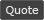WoWInterface - View Single Post - Changing Text Formatting
 Thread: Changing Text Formatting View Single Post
05-06-17, 10:09 PM   #2
SDPhantom
A Pyroguard Emberseer
Join Date: Jul 2006
Posts: 1,974
Here's what I've started doing with my number displays.
Lua Code:
`local math_abs=math.abs;local math_floor=math.floor;local math_log10=math.log10;local math_max=math.max;local tostring=tostring; local NumberCaps={"K","M","B","T"};local function AbbreviateNumber(val)    local exp=math_max(0,math_floor(math_log10(math_abs(val))));    if exp<3 then return tostring(math_floor(val)); end     local factor=math_floor(exp/3);    local precision=math_max(0,2-exp%3);    return ((val<0 and "-" or "").."%0."..precision.."f%s"):format(val/1000^factor,NumberCaps[factor] or "e"..(factor*3));end`

It dynamically adjusts the precision based on if the number is in the 1's, 10's, or 100's. It'll revert to scientific notation if the abbreviation accessed is out of the defined scope. You can add more in the NumberCaps table.
__________________